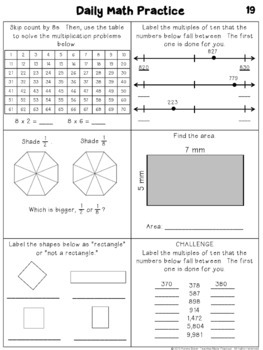# 3rd Grade Daily Math Practice / Math Morning Work: Quarter 23rd, Homeschool
Subjects
Standards
Resource Type
Formats Included
• PDF
Pages

#### Also included in

1. This no prep 3rd grade math review is ideal for morning work or daily math practice. It includes 180 days (4 quarters) worth of practice, enough for all year. The math skills are scaffolded, starting easier and getting progressively more difficult as the year progresses. Supports are in place thro
\$18.00
\$26.00
Save \$8.00

### Description

This no prep 3rd grade resource makes preparing math morning work or daily math practice easy. The math skills are scaffolded, starting easier and getting progressively more difficult as the quarter progresses. Supports are in place throughout the morning work to help students be able to complete the morning work / math review both independently and successfully.

Save over 30% when you buy 3rd Grade morning work / math practice for all year.

45 days (9 weeks) of daily math practice is included in this resource for the 2nd quarter of school. Every day, students will answer a math question from each of the 5 Common Core math domains for 3rd grade, as well as a challenge question.

Students will practice the following Common Core math standards throughout the quarter:

Operations and Algebraic Thinking: 3.OA.C.7

Fluently multiply and divide within 100, using strategies such as the relationship between multiplication and division (e.g., knowing that 8 × 5 = 40, one knows 40 ÷ 5 = 8) or properties of operations. By the end of Grade 3, know from memory all products of two one-digit numbers.

Number and Operations in Base 10: 3.NBT.A.1

Use place value understanding to round whole numbers to the nearest 10 or 100.

Number and Operations - Fractions: 3.NF.A.3

Explain equivalence of fractions in special cases, and compare fractions by reasoning about their size.

Measurement and Data: 3.MD.C.7.A, 3.MD.C.7.B

Find the area of a rectangle with whole-number side lengths by tiling it, and show that the area is the same as would be found by multiplying the side lengths.

Multiply side lengths to find areas of rectangles with whole-number side lengths in the context of solving real world and mathematical problems, and represent whole-number products as rectangular areas in mathematical reasoning.

Geometry: 3.G.A.1

Understand that shapes in different categories (e.g., rhombuses, rectangles, and others) may share attributes (e.g., having four sides), and that the shared attributes can define a larger category (e.g., quadrilaterals). Recognize rhombuses, rectangles, and squares as examples of quadrilaterals, and draw examples of quadrilaterals that do not belong to any of these subcategories.

This resource can be used for math morning work, daily math practice, or for homework.

Save 30% when you buy the math practice for the entire year, or buy each quarter separately:

1st Quarter Morning Work

3rd Quarter Morning Work

4th Quarter Morning Work

Total Pages
Included with rubric
Teaching Duration
2 months
Report this Resource to TpT
Reported resources will be reviewed by our team. Report this resource to let us know if this resource violates TpT’s content guidelines.

### Standards

to see state-specific standards (only available in the US).
Understand that shapes in different categories (e.g., rhombuses, rectangles, and others) may share attributes (e.g., having four sides), and that the shared attributes can define a larger category (e.g., quadrilaterals). Recognize rhombuses, rectangles, and squares as examples of quadrilaterals, and draw examples of quadrilaterals that do not belong to any of these subcategories.
Explain equivalence of fractions in special cases, and compare fractions by reasoning about their size.
Fluently multiply and divide within 100, using strategies such as the relationship between multiplication and division (e.g., knowing that 8 × 5 = 40, one knows 40 ÷ 5 = 8) or properties of operations. By the end of Grade 3, know from memory all products of two one-digit numbers.
Use place value understanding to round whole numbers to the nearest 10 or 100.
Multiply side lengths to find areas of rectangles with whole-number side lengths in the context of solving real world and mathematical problems, and represent whole-number products as rectangular areas in mathematical reasoning.